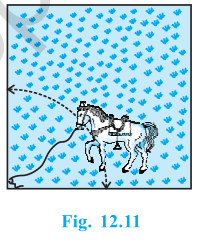# A horse is tied to a peg at one corner of a square shaped grass field of side 15 m by means of a 5 m long rope (see Fig.) . Find (i) the area of that part of the field in which the horse can graze. (ii) the increase in the grazing area if the rope were 10 m long instead of 5 m. (Use π = 3.14)

As the horse is tied at one end of a square field, it will graze only a quarter (i.e. sector with θ = 90°) of the field with radius 5 m.(i) Area of circle = πr= 22/7 × 52 = 78.5 m2

The area of the part of the field where the horse can graze

= ¼ (the area of the circle)

= 78.5/4

= 19.625 m2

(ii) If the rope is increased to 10 m,

Area of circle will be = πr2 =22/7×102 = 314 m2

Now, the area of the part of the field where the horse can graze

= ¼ (the area of the circle)

= 314/4

= 78.5 m2

∴ Increase in the grazing area = 78.5 m2 – 19.625 m2 = 58.875 m2